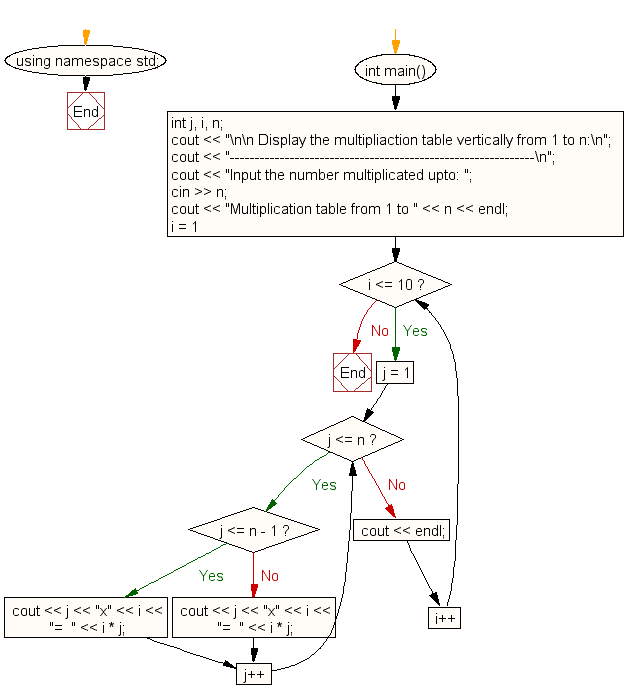﻿ C++ : Multiplication table vertically from 1 to n# C++ Exercises: Display the multiplication table vertically from 1 to n

## C++ For Loop: Exercise-19 with Solution

Write a program in C++ to display the multiplication table vertically from 1 to n.

Sample Solution:-

C++ Code :

``````#include <iostream>
using namespace std;

int main()
{
int j, i, n;

cout << "\n\n Display the multipliaction table vertically from 1 to n:\n";
cout << "-------------------------------------------------------------\n";
cout << "Input the number upto 5: ";
cin >> n;

cout << "Multiplication table from 1 to " << n << endl;
for (i = 1; i <= 10; i++)
{
for (j = 1; j <= n; j++)
{
if (j <= n - 1)
cout << j << "x" << i << "=  " << i * j;
else
cout << j << "x" << i << "=  " << i * j;
}
cout << endl;
}
}
``````

Sample Output:

``` Display the multipliaction table vertically from 1 to n:
-------------------------------------------------------------
Input the number multiplicated upto: 5
Multiplication table from 1 to 5
1x1=1  2x1=2  3x1=3  4x1=4  5x1=5
1x2=2  2x2=4  3x2=6  4x2=8  5x2=10
1x3=3  2x3=6  3x3=9  4x3=12  5x3=15
1x4=4  2x4=8  3x4=12  4x4=16  5x4=20
1x5=5  2x5=10  3x5=15  4x5=20  5x5=25
1x6=6  2x6=12  3x6=18  4x6=24  5x6=30
1x7=7  2x7=14  3x7=21  4x7=28  5x7=35
1x8=8  2x8=16  3x8=24  4x8=32  5x8=40
1x9=9  2x9=18  3x9=27  4x9=36  5x9=45
1x10=10  2x10=20  3x10=30  4x10=40  5x10=50
```

Flowchart:C++ Code Editor:

Contribute your code and comments through Disqus.

What is the difficulty level of this exercise?

﻿

## C++ Programming: Tips of the Day

What is a smart pointer and when should I use one?

This answer is rather old, and so describes what was 'good' at the time, which was smart pointers provided by the Boost library. Since C++11, the standard library has provided sufficient smart pointers types, and so you should favour the use of std::unique_ptr, std::shared_ptr and std::weak_ptr.

There was also std::auto_ptr. It was very much like a scoped pointer, except that it also had the "special" dangerous ability to be copied - which also unexpectedly transfers ownership.

It was deprecated in C++11 and removed in C++17, so you shouldn't use it.

```std::auto_ptr<MyObject> p1 (new MyObject());
std::auto_ptr<MyObject> p2 = p1; // Copy and transfer ownership.
// p1 gets set to empty!
p2->DoSomething(); // Works.
p1->DoSomething(); // Oh oh. Hopefully raises some NULL pointer exception.
```

Ref : https://bit.ly/3mc9GHE